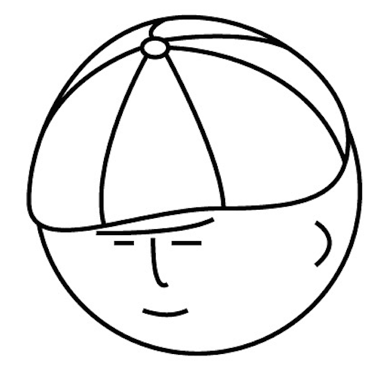# マツシタのお勉強メモ

#### マツシタのお勉強

##### welcome to my engineering blog
###### Hacker Rank# Binary Indexed Tree (BIT)

Binary Indexed Tree makes it possible to implement the following queries with O(log N).

1. To calculate sum of values in range
2. To update the value of index

#### Basic idea

This structure is Binary Tree like Segment Tree. But BIT is much easier to code, and require less memory space than RMQ.

Difference between Segment Tree and BIT is that In BIT case, unnecessary section can be taken. So, we can save much memory and make it faster to operate above the queries.

# How to Implement

How to implement is like this.

#### Update Value

We can find the indexes to update by sum of index and the length of section.

#### Sum of Values

We can find the indexes to add by subtracting the length of section from index.

#### How to calculate the length of section

We can calculate the length by operating bit.

`length = (i & -1)`